# Thesis – Appendix A: Wavelet Analysis to Calculate Power Spectra

### Wavelet Analysis to Calculate Power Spectra

#### Overview

Wavelet analysis techniques, while not as commonly understood as Fourier analysis, are nonetheless frequently applied to problems in which time and frequency information are desired simultaneously. Analysis suites such as IDL (popular in plasma physics circles) provide complete libraries for easily incorporating these techniques into a research program. One of the best introductory pieces on wavelet analysis [Torrence and Compo, 1998], serves as the foundation for the IDL implementation (see notes in source file wv_cwt.pro, a routine used for the analysis presented here).

It is not the aim of this thesis to reproduce basic introductory material that is readily available, so the details of wavelet analysis are left to the many textbooks and other materials that exist. In simplified terms, a wavelet analysis is the application of a bandpass filter with logarithmic spacing in the frequency domain. The discussion in this Appendix concentrates on comparison to and validation against the more well established Fourier techniques that can be applied to the same data.

A wavelet as a function of time, Ψ(t), is defined as (Eq. 6.1.4 of Debnath ), (Eq. A.1),$\Psi(t) = \frac{1}{\sqrt{a}} \Psi\left( \frac{t - b}{a} \right)$

where a is a scaling parameter that sets the frequency represented by the wavelet and b determines the time center of the wavelet. The Continuous Wavelet Transform (CWT), W, of a function of time, f(t), is (Eq. 6.2.4 of [Debnath, 2002]), (eq. A.2)$W(f) = \int_{-\infty}^{+\infty} f(t) \overline{\Psi(t)} dt$

where the scale and time center is still determined by the a and b parameters of the wavelet. The wavelet power spectrum, PW, is therefore given by PW = |W(f)|2.

A full spectrogram is generated through wavelet analysis by setting the scale (a) to a constant value and solving across all time values (b). Repeating this process for all scales that translate to a relevant frequency completes the analysis.

#### Parameters of Wavelet Analysis

The description of wavelet analysis as a logarithmically spaced comb filter (i.e., picking out the power in a specific, non-continuous array of frequencies) easily incorporates the concept of basis functions. The basis function is the specific filter used in the analysis. Wavelet basis functions come in a wide variety and it is left to the user to determine the best option for any given analysis. This is a difficulty in wavelet analysis that has no analog in Fourier analysis because that transformation is unique.

Wavelet basis functions can be real or complex valued, discrete or continuous, and orthogonal or non-orthogonal. The turbulence study presented here depends on the availability of phase analysis, which immediately requires that only complex valued basis functions be used. The ability to review specific ranges of frequencies is also necessary, suggesting the use of continuous bases. These two desired properties are the most important features to consider. The primary difference between the orthogonal property choices of a wavelet basis is that a non-orthogonal basis will “double-count” the power contribution of some frequencies, thereby returning inaccurate absolute amplitudes. The basis ultimately chosen for this work, the Morlet wavelet, is non-orthogonal. The potentially inaccurate amplitudes of the power spectra are overcome by only applying this technique to study the temporal behavior of modes and not their spatial structure or other features that rely on amplitude measures.

The Morlet wavelet, ΨM, is written as (Eq. 6.2.12 of [Debnath, 2002]), (Eq. A.3),$\Psi_M = \exp\left( i\omega_0t - \frac{t^2}{2} \right)$

where ωo is a variable describing the frequency at which the Fourier transform of the Morlet wavelet demonstrates peak amplitude.

To review the behavior of wavelets used in various analyses it is helpful to utilize test signals. Figure A.1 is a plot of pure sine waves of 5 kHz (top) and 50 kHz (bottom). Both signals are centered on zero for the spectral analysis and only offset to provide for clear review in the figure.

The 5 kHz test signal is used to highlight the frequency resolution of the Morlet wavelet. Figure A.2 compares the power spectra of the 5 kHz test signal for three wavelet basis functions: Morlet, Gaussian, and Paul. The Gaussian and Paul wavelet return similar results that exhibit poor frequency resolution compared to the Morlet. This early benchmarking of wavelet performance is necessary because of the numerous options in setting the parameters of the analysis. Deciding on the Morlet basis is relatively simple, but it remains to compare the Morlet wavelet results to those computed with standard Fourier methods.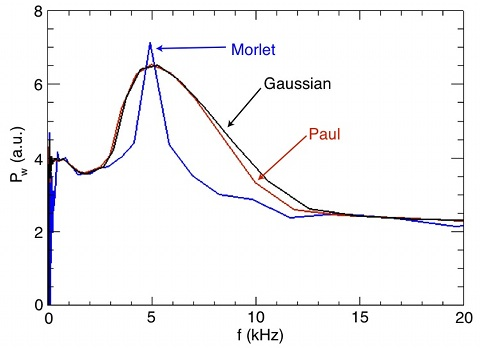Figure A.2: Wavelet power spectra to identify the test signal’s 5 kHz mode using different wavelet basis functions. The Morlet wavelet results in the best frequency resolution.

#### Fixed Frequency Test Signals

The most significant difference between a wavelet spectrum and that of an FFT is that the wavelet technique results in a bandwidth that is frequency dependent. The bandwidth, or frequency resolution, of an FFT is determined by the total length of the input, l. The frequency resolution is constant and given by Δf = 1/l where l is the time length of the data window over which the FFT is performed. For a time series of N data points, output power spectrum provides a value for every frequency from f = 0 to f = (N/2)(1/l).

Wavelet analysis returns spectra in which the value of Δf/f is constant [Farge, 1992]. For an FFT, Δf/f decreases with increasing frequency because Δf is constant. In a wavelet result, the resolution of higher frequency terms is worse than that of lower frequencies. An illustration of this is shown by analyzing the test signals of Fig. A.1.

Figure A.3 plots the resulting power spectra from performing transforms using either FFT or Continuous Wavelet Transform (CWT) techniques. Peaks in the FFT spectra accurately identify the center frequencies of the test signals. The peak in the 5 kHz result (green) is just as narrow as the peak corresponding to the 50 kHz result (blue). Peaks from the CWT analysis demonstrate a reduction in frequency resolution for the higher frequency wave. The ratio of the frequency spacing to the center frequency is constant for the CWT result. Figure A.3, can be used to calculate these values, (Eq. A.4 for 5 kHz)$\frac{\Delta f}{f} = \frac{2.026}{5} = 0.405$

(Eq. A.5 for 50 kHz),$\frac{\Delta f}{f} = \frac{18.91}{50} = 0.378$

This demonstrates that wavelet techniques are comparable to Fourier techniques for calculating power spectra. In the context of this thesis, wavelet analysis is used to provide a broad picture of the time evolved spectra. Any determination of a particular mode frequency is done with Fourier analysis in order to more precisely determine the value.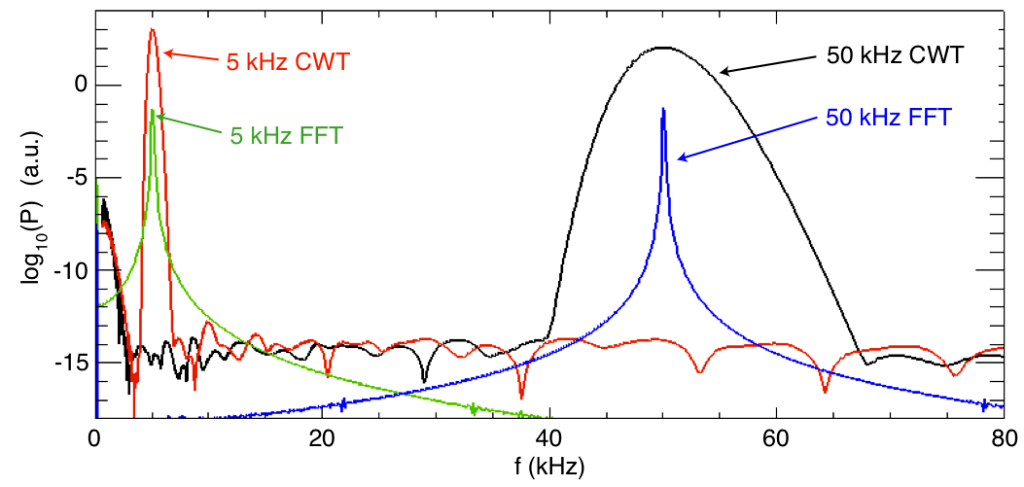Figure A.3: Power spectra of the test signals. The FFT analysis is clearly peaked at the frequencies of the test signals. The CWT results provide a less clear peak for the higher frequency signal.

#### Evolving Frequency Test Signals

Figure A.4 is a narrow time view of the test signal used to demonstrate the ability of the wavelet analysis technique to discern the temporal behavior of coherent modes. This signal is a regular 5 kHz sine wave onto which a 17 kHz sine is added, beginning at t ≈ 5.25 ms. A spectrogram of this test signal is expected to reveal the constant presence of the 5 kHz and the sudden onset of the 17 kHz oscillation.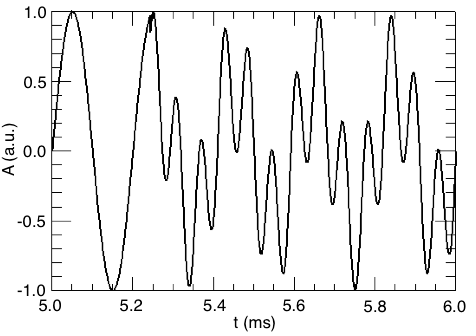Figure A.4: The amplitude, A, of the test signal featuring a secondary frequency. This narrow time region of the complete signal highlights the activation of a 17 kHz signal at t ≈ 5.25 ms. The background, constant, oscillation is a 5 kHz wave.

Figure A.5 is a wavelet spectrogram of this test signal. The constant 5 kHz wave is identified throughout the entire time. The exact turn-on of the 17 kHz mode is difficult to discern based on the broad time range returned. It is noteworthy that the time resolved nature of the sudden turn-on is given accurately by the higher frequency behavior. The immediate injection of the 17 kHz signal carries with some type of &delta-function influence that causes power to be calculated for all frequencies. In this way, any sudden event in a time series can be determined to reasonable accuracy through the wavelet method, regardless of any particular frequency that may be associated with the phenomenon.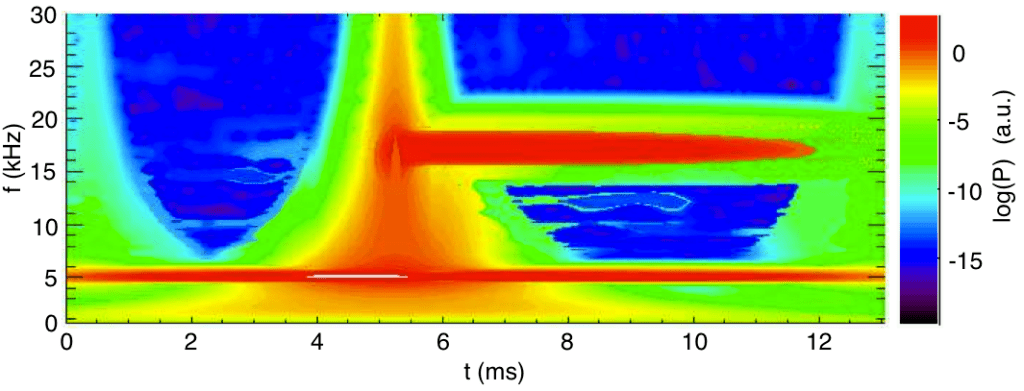Figure A.5: Spectrogram calculated using the CWT technique on the evolving frequency test signal. The appearance of the 17 kHz oscillation is evident, though the best time resolution for marking this abrupt change comes at higher frequencies.

#### Experimental Data

Figure A.6 presents spectrograms (power spectra over time) from the temperature filament experiment that demonstrate the differences between CWT and FFT analyses. These spectra are calculated from measurements of electron temperature in the outer region of the filament in an experiment for which the background magnetic field is Bo = 900 G.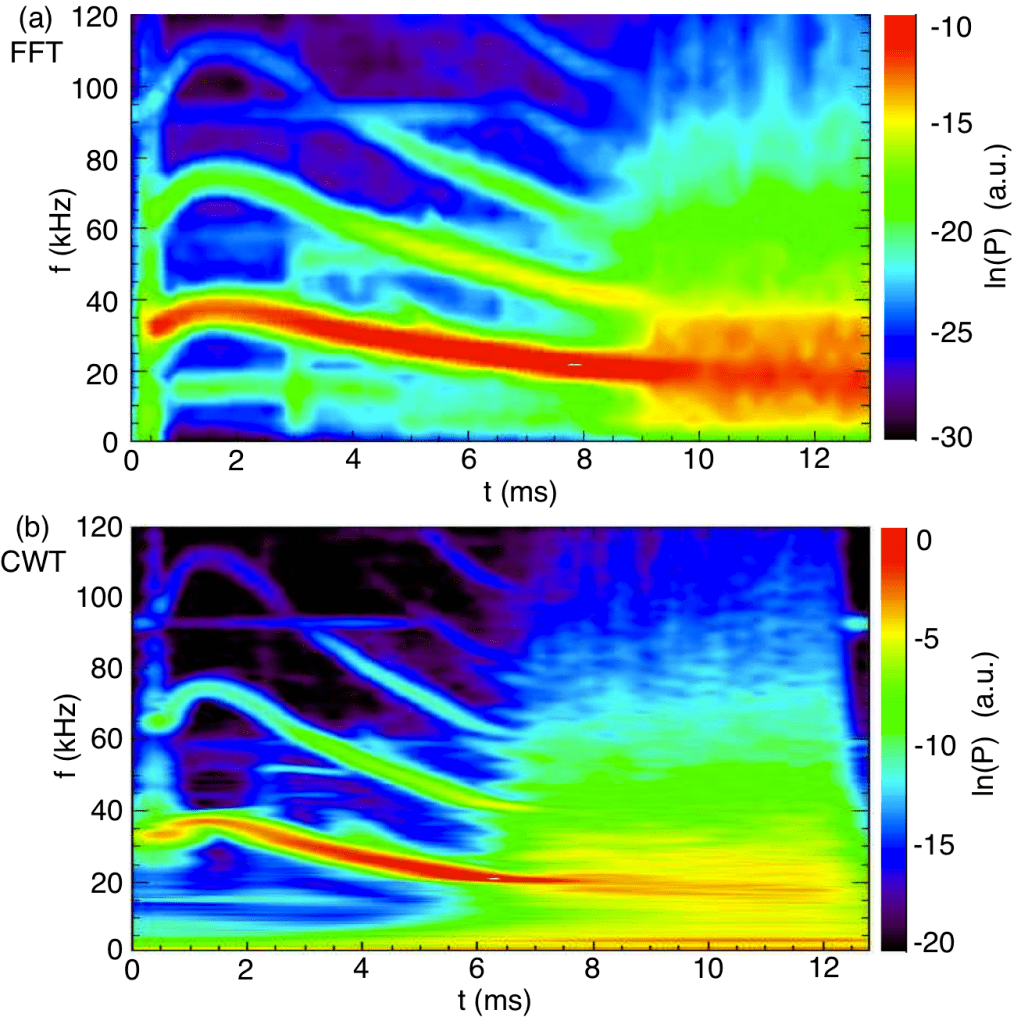Figure A.6: (a) Spectrogram calculated using a sliding FFT window to compute the spectrum for individual times. The central time of the FFT window is used to position the result. The transition to broadband turbulence appears as a sharp event at t = 9 ms. (b) Spectrogram calculated using the CWT method. The transition to turbulence appears as a process beginning near t = 7 ms.

In Fig. A.6a a coherent drift-Alfvén mode (f ≈ 30 kHz) and its harmonics is observed to Doppler shift during the first 9 ms of the temporal evolution. This same mode is clearly visible in the CWT representation of Fig. A.6b. Any differences in frequency resolution between these methods is insignificant. In fact, the CWT results in a seemingly better identification of mode frequency for the drift-Alfvén wave. The delay between time zero (beginning of beam heating) and the appearance of features within the spectrograms is due to the time it takes for these features to appear at the measurement position 256 cm axially separated from the beam.

The most striking difference between these two results concerns the temporal features of the transition to broadband spectra. The FFT result of Fig. A.6a shows a sharp transition from the coherent mode to a broadband spectra at t = 9 ms. The CWT result of A.6b indicates that this transition may not be as dramatic and that it begins earlier than t = 9 ms. The sharp transition shown in the FFT spectrogram results from the windowing method employed. The windowed FFT computes a handful of individual spectra over user-defined time regions of the input time series. The larger the time window used, the better the frequency resolution of the spectra according to the Δf relation given earlier. Improved frequency is achieved by reducing temporal resolution. The sharp transition in the FFT spectrogram appears because one window covers none of the broadband fluctuations while the next window is the first one to detect them. The window that first detects them returns a significantly different result from the preceding window. The CWT method does not window across the data and therefore provides a better temporal resolution in this case (the poor frequency resolution at high frequencies is the tradeoff for this method). It is generally the case that a combination of FFT and CWT techniques provides the most complete analysis of spectral features in an experiment.